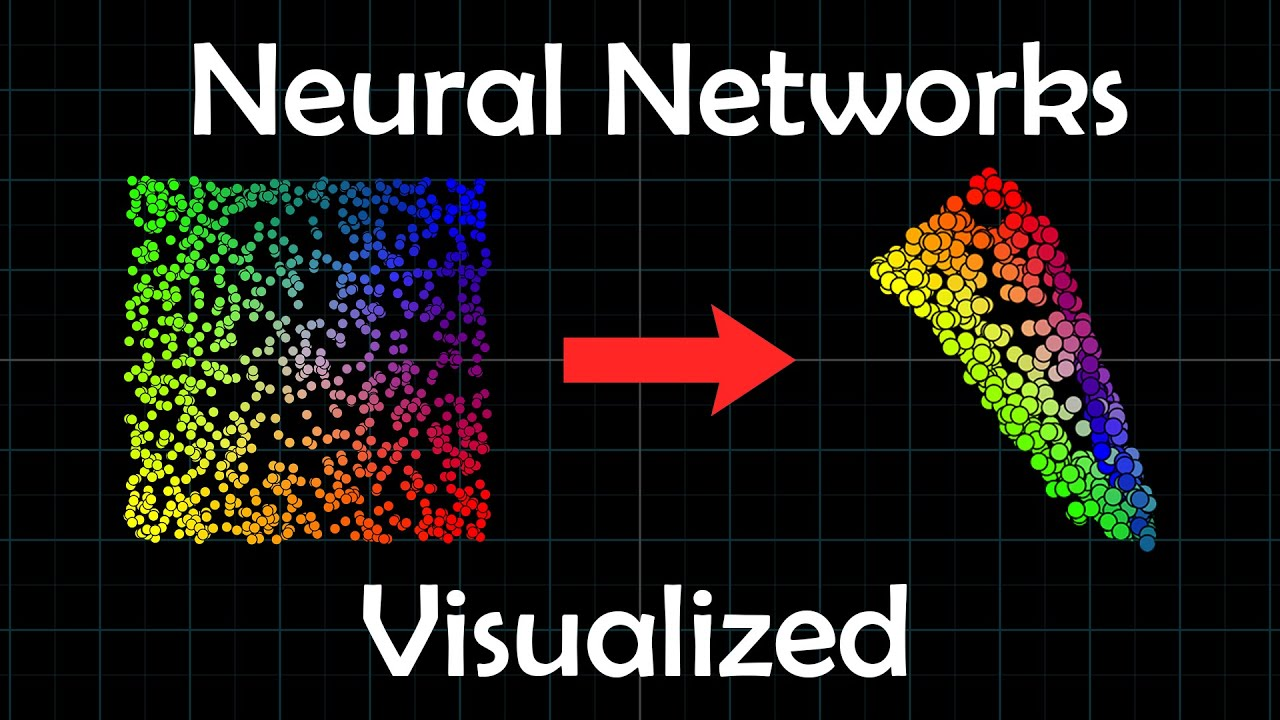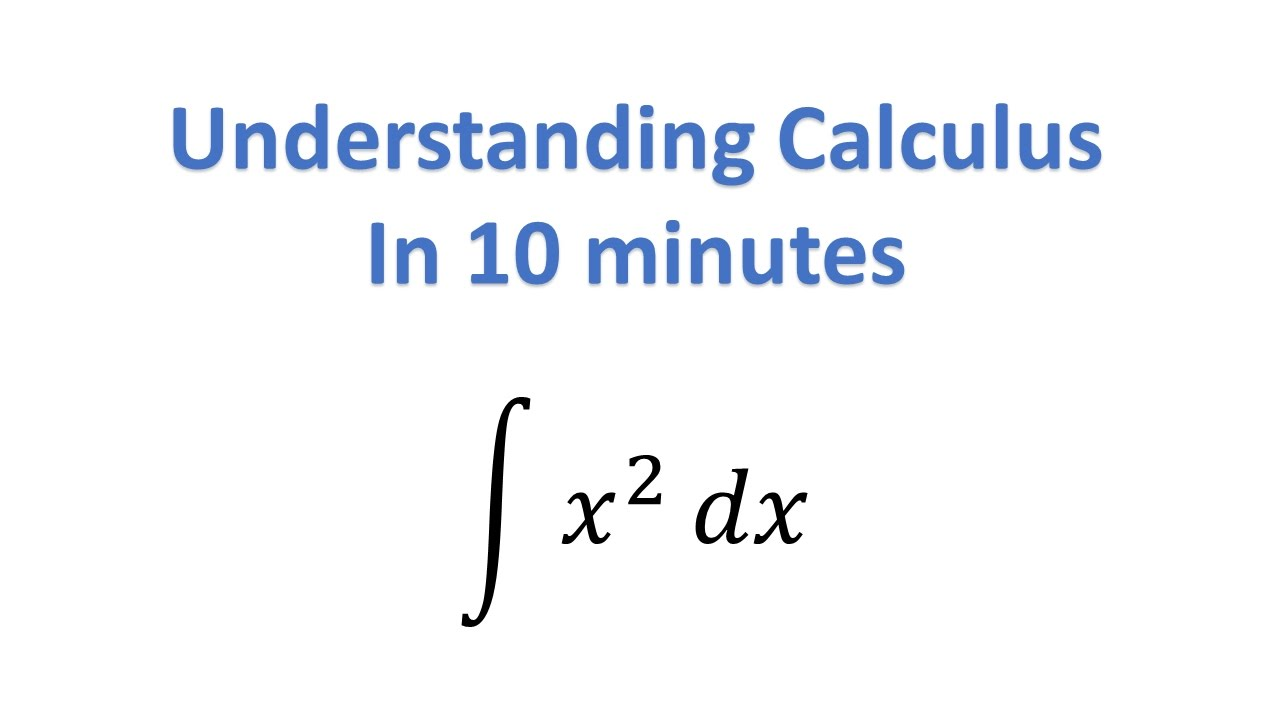# derivativeMathematics

#### 3 Paradoxes That Gave Us Calculus

Up and Atom examines the three paradoxes that produced calculus.Data Visualization Neural Networks

#### Visualizing Deep Learning

vcubingx provides a visual introduction to the structure of an artificial neural network. The Neural Network, A Visual Introduction | Visualizing Deep Learning, Chapter 1 0:00 Intro 1:55 One input Perceptron 3:30 Two input Perceptron 4:40 Three input Perceptron 5:17 Activation Functions 6:58 Neural Network 9:45 Visualizing 2-2-2 Network 10:59 Visualizing 2-3-2 Network 12:33 Classification […]Funny Mathematics

#### Calculus Rhapsody

In honor or Pi Day, here is Calculus Rhapsody.